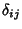## Scale Factor

For a diagonal Metric Tensor, whereis the Kronecker Delta, the scale factor is defined by(1)

The Line Element (first Fundamental Form) is then given by(2)(3)

The scale factor appears in vector derivatives of coordinates in Curvilinear Coordinates.